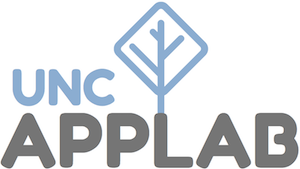# A Clojure Tutorial

Did you know that there is a programming language that’s not only consistently enjoyable to use, but also amazingly productive—and which was the highest paying language according to the 2018 Stack Overflow developer survey? In this talk, Professor of the Practice and App Lab director Jeff Terrell introduces the programming language Clojure.

Watch it on YouTube

Topics:

• Why Clojure? (0:00)
• Basic syntax and literals (7:06)
• Basic built-in functions (20:55)
• `let` blocks (28:17)
• Defining variables and functions (34:35)
• Immutability (35:59)
• Data structures as functions (40:45)
• Clojure philosophy: bring data to the center (with HTML and HTTP examples) (45:37)
• Overview of other cool Clojure things (56:54)
• Where to go from here (61:40)

You can see the notes for the talk here. These notes are valid clojure code and you can run them and modify them on your computer. You can run these in a repl on your computer or, if you haven’t installed clojure, in a Clojure repl on Repl.it.

``````(ns user
(:require
[clojure.repl :refer :all]))
​
;; 0. Why Clojure?
;;    - joy
;;    - elegance and simplicity
;;    - high paying jobs
;;    - mind expansion
​
;; 1. Basic syntax
1            ; number literal
1/3          ; fractions
1N           ; big ints (clojure.lang.BigInt)
3.14159M     ; big decimals (java.math.BigDecimal)
"string"     ; string literal
:keyword     ; keyword
;foo         ; symbol; evaluates to the value of foo
(list 1 2 3) ; list
[1 2 3]      ; vector
{:foo 7}     ; hashmap
(+ 1 2)      ; function call: function then arguments
(+ 1 2 3 4)  ; arbitrary number of arguments to some functions
,            ; whitespace (really!)
;foo+bar, foo&bar, foo->bar, foo?, bar!  ; legal symbol names
#(+ 5 %)     ; function literal: add 5 to the given argument
(if true "true" "false")  ; conditional
​
;; 2. Basic built-in functions
(range 5)
(inc 7)
(dec 7)
(doc dec)
(map inc (range 5))
(filter even? (range 5))
(map #(* % %) (range 5))
(reduce + 0 (range 5))
(str "Join " "multiple " "things " 1 :foo  " as a string.")
(-> 7 inc inc inc)
(-> 7 inc (/ 2) (+ 4))
(->> 3 dec (/ 2))
;; sum of the first 10 odd squares
;; also demonstrates laziness
(->> (range)
(map #(* % %))
(filter odd?)
(take 10)
(reduce + 0))
​
;; 3. let blocks
(let [square #(* % %)
nonneg-ints (range)
squares (map square nonneg-ints)
odd-squares (filter odd? squares)
first-10 (take 10 odd-squares)
sum (reduce + 0 first-10)]
sum)
(let [foo 7
foo (* foo foo)]
(inc foo))
​
;; 4. Defining variables and functions
(def v [9 1])
v  ; symbols evaluate to the value they reference
(defn square
"Return the square of the given number."
[x]
;; implicit return
(* x x))
(square 7)
(map square (range 5))
(def square2 (fn [x] (* x x)))
((fn [x] (* x x)) 7)
​
;; 5. Immutability
;; - immutability and ways to use it (e.g. update, sum a list of numbers)
(conj v 9)
v  ; unchanged!
(subvec v 1 2)
v  ; unchanged!
(def hm {:foo 1, :bar 2})
hm
(assoc hm :baz 3)
hm
(merge hm {:yah 4})
hm
(dissoc hm :bar)
hm
(update hm :bar inc)
hm
​
;; 6. data structures as functions
(hm :foo)
(:foo hm)
(let [nickname {"Jeffrey" "Jeff"
"Jessica" "Jess"}]
(map nickname ["Jessica" "Jeffrey" "Johann"]))
(let [jeff {:name "Jeff", :age 37, :adult? true }
jane {:name "Jane", :age  4, :adult? false}
people [jeff jane]]
(let [jeff {:name "Jeff", :age 37, :adult? true }
jane {:name "Jane", :age  4, :adult? false}
people [jeff jane]]
(map :age people))
​
;; 7. make data & transformations the essence of your program
(comment
(require '[ring.adapter.jetty :refer [run-jetty]])
(require '[clojure.pprint :refer [pprint]])
(defn handler
[request]
(pprint request)
{:status 204})
(run-jetty handler {:port 3003})
)
​
(comment
(require '[hiccup.core :refer [html]])
(html
[:ul#id.class {:attr "value"}
[:li "yo"]])
(let [li (fn [index] [:li (str "Item #" (inc index))])
lis (map li (range 5))]
(html [:ul lis]))
)
​
;; 8. other cool things
;; - Java interop, JVM runnability
;; - Clojurescript, compiles to Javascript; js interop, re-frame, etc.
;; - Clojure syntax, pros and cons (macros, structural editing) (TODO: demo?)
;; - Datomic
​
;; 9. where to go from here
;; - Install Clojure: https://applab.unc.edu/posts/2019/09/11/how-to-install-clojure-on-windows/
;; - Clojure cheatsheet: https://clojure.org/api/cheatsheet
;; - Clojure docs: https://clojuredocs.org/
;; - 4Clojure: http://www.4clojure.com/
​
;; 10. Exercises (from 4Clojure):
​
;; - Define a function that computes factorials. You may assume the argument is
;;   a non-negative integer. Define your function as !. (Useful functions: = *)
​
(read-string "(+ 1 2)")
​
(comment
(= (! 1) 1)
(= (! 3) 6)
(= (! 5) 120)
(= (! 8) 40320))
​
;; - Write a function which takes a vector of integers as an argument. Return
;;   the count of how many elements are smaller than the sum of their squared
;;   component digits. For example: 10 is larger than 1 squared plus 0 squared;
;;   whereas 15 is smaller than 1 squared plus 5 squared. Define your function
;;   as f. (Useful functions: < quot rem)
​
(comment
(= 8 (f (range 10)))
(= 19 (f (range 30)))
(= 50 (f (range 100)))
(= 50 (f (range 1000))))
​
(comment
(defn ->hhmm [s]
(format "%d:%02d"
(quot s 60)
(rem s 60)))
(->hhmm 3700)
)``````